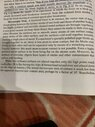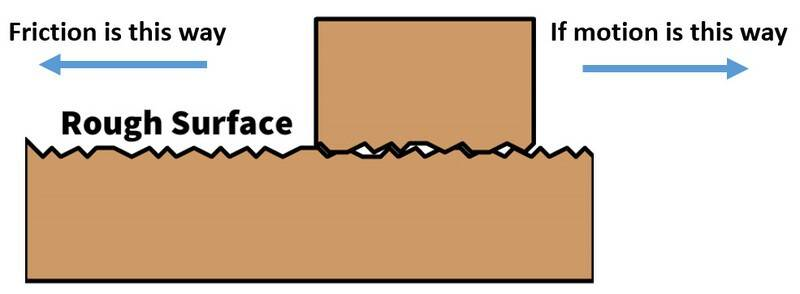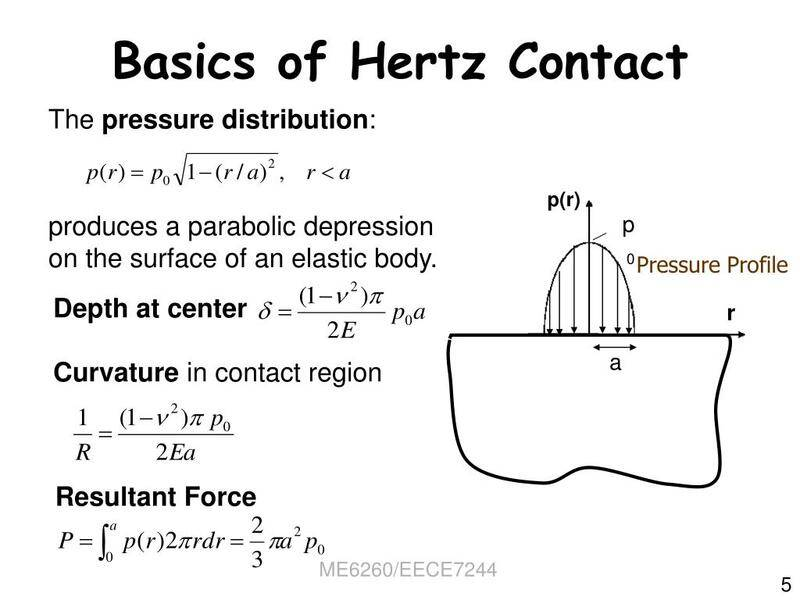# Reason behind friction

Gold Member
I read friction is the vector sum of the forces acting between the atoms of one surface and another. But the direction of friction is parallel to surface. I don’t get how friction occurs.
I will also add the paragraph from my book “resnik” for further clarification.

Other question is ##{f_{s,max}}={{mu}_s}{F_N}##.
Can it also be applied force in place of normal force? Can I write the eqn with applied force in it? ##{f_{s}}## is proportional to applied force too.

#### Attachments

•image.jpg
67.3 KB · Views: 32
Last edited:

But the direction of friction is horizontal.
Not horizontal, but parallel to the surface.
Can it also be applied force in place of normal force?
That is not how the friction coefficient is defined.

Homework Helper
Gold Member
Note how the book clarifies that the forces acting between the atoms of two surfaces in all directions only happens where those atoms can be close enough, which total surface could be around 10,000 times smaller than the geometric contact area.

https://www.texasgateway.org/resource/51-friction?book=79096&binder_id=78531Gold Member
I don’t get how friction occurs.

Below you have an image showing what two surfaces in contact with each other look like on a microscopic level. The small peaks are constantly broken as the parts move, thus the friction force. The rougher they are, the higher the friction force.•sysprog
Mentor
If you want to learn more about friction, a good search term is tribology, and this is a good place to start: https://www.stle.org/files/About_STLE/Tribology/files/What_is_tribology/Tribology.aspx.

Be advised that friction is a field of study where you can spend a lifetime learning, and still not know it all. A quote from the above link: The field is necessarily interdisciplinary and utilizes skills from mechanical engineering, materials science and engineering, chemistry and chemical engineering and more. Or you can learn that friction force is the friction coefficient multiplied by the normal force, how to apply that relationship, and stop there.

•russ_watters, rudransh verma and sysprog
Gold Member
Note how the book clarifies that the forces acting between the atoms of two surfaces in all directions only happens where those atoms can be close enough, which total surface could be around 10,000 times smaller than the geometric contact area.

https://www.texasgateway.org/resource/51-friction?book=79096&binder_id=78531“So Coefficient of friction is a unitless quantity with a magnitude usually between 0 and 1.0. The coefficient of the friction depends on the two surfaces that are in contact”
Q1. But what is the meaning of this coefficient? Is it not related to area of surface?
Ans: No!

“We have noted that friction is proportional to the normal force but not to the area in contact, a somewhat counterintuitive notion. When two rough surfaces are in contact, the actual contact area is a tiny fraction of the total area since only high spots touch. When a greater normal force is exerted, the actual contact area increases, and it is found that the friction is proportional to this area.”

Q2.What is this molecular interaction actually and the forces acting between the atoms of two surfaces in all directions ?
Is it electrical ?
Ans: yes!

#### Attachments

Last edited:
Gold Member
Q1. But what is the meaning of this coefficient?
It means that the friction force is proportional to the normal force. It is just a representation of what we can observe.
Is it not related to area of surface?
You could rewrite the equation in terms of the area ##A## and the normal pressure ##P_N##:
$$f_{s,max}=\mu_s P_NA$$
Where ##P_N = \frac{F_N}{A}##.

Does that make more sense? What it means is that when you increase the area you give more surface for the normal force to act - thus creating more friction - but at the same time you also divide the normal force into smaller forces acting locally. Both effects cancel each other out.
Q2.What is this molecular interaction actually and the forces acting between the atoms of two surfaces in all directions ?
Is it electrical ?
Atoms tend to stick together. The strength of these bonds - among other things - can define their state (solid, liquid, or gas). You put hydrogen and oxygen atoms together and, in the right conditions, you get water, i.e. hydrogen and oxygen atoms strongly sticking together.

Friction is a type of weak molecular bond that exists between atoms.

Gold Member
Friction is a type of weak molecular bond that exists between atoms.
Like inter molecular electric forces between +H and -O of two water molecules.
Edit: so it’s not the hills and valleys of the surface or is it the combination of both?

1.And if we place a weight on top of a box then due to increase in normal force and pressing into the surface more and more part of rough surface come in contact creating more friction.

2.And as we increase the area of the box then again this friction increases since more surface area comes in contact. But it doesn’t directly depend on area but on the smoothness and roughness of the surfaces.

3.Also friction doesn’t directly depend on applied force but on the smoothness/roughness of area and weight of the body.

4. If too much smooth are surfaces then they mend into one known as cold welding.

5. And my last question is that is ##mu## a measure of force of adhesion ?

Pls Verify !

Last edited:
Homework Helper
Like inter molecular electric forces between +H and -O of two water molecules.

For me, whether it is a Van Der Waals bond being created and then broken between nearby molecules or whether it is microscopic ridges bumping and bouncing chaotically from one another is irrelevant. Friction (##F_f=\mu F_N##) is an engineering approximation describing the behavior between two surfaces in contact.
1.And if we place a weight on top of a box then due to increase in normal force and pressing into the surface more and more part of rough surface come in contact creating more friction.
That is one physical explanation for the correctness of the engineering approximation.
2.And as we increase the area of the box then again this friction increases since more surface area comes in contact. But it doesn’t directly depend on area but on the smoothness and roughness of the surfaces.
If you increase surface area while holding contact force constant, the approximation says that frictional force remains constant. The truth is that the approximation is good, but still only approximate. The frictional force may change slightly with increased surface area.
3.Also friction doesn’t directly depend on applied force but on the smoothness/roughness of area and weight of the body.
Friction does depend directly on the applied force. Whether that force is from the weight of the sanding block on the floor or the force of the fellow pushing the sanding block into the wall does not matter.
4. If too much smooth are surfaces then they mend into one known as cold welding.
If you are trying to understand friction then you should not be trying to understand it in terms of cold welding.
5. And my last question is that is ##mu## a measure of force of adhesion ?
The engineering approximation says that friction is proportional to normal force. ##\mu## is simply the constant of proportionality.

•Merlin3189
Gold Member
If you increase surface area while holding contact force constant, the approximation says that frictional force remains constant.
You mean the weight should also increase with area for increase in friction. Right!
The engineering approximation says that friction is proportional to normal force. μ is simply the constant of proportionality.
When friction changes is it because of the mu that changes with changing applied force? Something like that?
This muk < mus always because Fk< Fs . To keep the body moving with uniform speed we need to decrease the applied force to Fk from Fsmax.

Homework Helper
You mean the weight should also increase with area for increase in friction. Right!

When friction changes is it because of the mu that changes with changing applied force? Something like that?
This muk < mus always because Fk< Fs . To keep the body moving with uniform speed we need to decrease the applied force to Fk from Fsmax.
The ##\mu## is (ideally) a constant for any particular pair of surfaces. It does not change (much).

To keep a body moving with uniform speed, we need to match the applied tangential force with ##\mu## times the applied normal force. If the normal force is increased, the tangential force will have to increase or the body will slow down. If the normal force is decreased, the tangential force will have to decrease or the body will speed up. ##F_k = \mu F_n##.

The force of static friction and the coefficient of static friction have nothing whatsoever to do with keeping the sanding block moving at a constant pace. If it is already moving... then it is already moving.

Gold Member
The force of static friction and the coefficient of static friction have nothing whatsoever to do with keeping the sanding block moving at a constant pace. If it is already moving... then it is already moving.
Yeah! Kinetic friction has to do with keeping the body at constant pace.

I got it. So mu is simply a measure of the material of the two surfaces. Depending on the material mu varies. Different materials have different wander wal forces and roughness. This is what mu measures. And thus friction depends on two things. Material and normal force.

Last edited:
•jbriggs444
ergospherical
I think it is important to emphasise that the static friction does not satisfy ##F = \mu N##, but rather ##F \leq \mu N## with equality only holding when the surfaces are on the verge of slipping.

•rudransh verma
Gold Member
You could rewrite the equation in terms of the area A and the normal pressure PN:
fs,max=μsPNA
Where PN=FNA.

Does that make more sense? What it means is that when you increase the area you give more surface for the normal force to act - thus creating more friction - but at the same time you also divide the normal force into smaller forces acting locally. Both effects cancel each other out.
I don’t understand the sentence in bold. The final eqn doesn’t contain A. Mathematically friction doesn’t depend on Area as does @jbriggs444 noted. May vary a little bit.
But here is a paragraph to be considered:
“illustrates one macroscopic characteristic of friction that is explained by microscopic (small-scale) research. We have noted that friction is proportional to the normal force but not to the area in contact, a somewhat counterintuitive notion. When two rough surfaces are in contact, the actual contact area is a tiny fraction of the total area since only high spots touch. When a greater normal force is exerted, the actual contact area increases, and it is found that the friction is proportional to this area.”
I think this will be the explanation for why friction increases with normal force.

And mu is simply a measure of the material of the two surfaces. Depending on the material mu varies. Different materials have different wander wal forces and roughness. This is what mu measures. And thus friction depends on two things. Material and normal force.

Last edited:
Gold Member
I don’t understand the sentence in bold. The final eqn doesn’t contain A. Mathematically friction doesn’t depend on Area as does @jbriggs444 noted. May vary a little bit.
Say you have a weightless block with a surface area ##A## and a weight ##W## sitting on it. Let us assume the coefficient of friction is 1.0 and that the friction force is proportional to the weight and area. Thus the friction force is ##WA##.

Let's put the same weight ##W## on two of those blocks ##A##, sitting side-by-side. Now the normal force on each block is ##\frac{W}{2}## leading to a friction force ##\frac{W}{2}A## for each block. Since you have two blocks, the total friction force is ##\frac{W}{2}A + \frac{W}{2}A = WA##. So even if we double the area by putting an extra block, we also are halving the forces acting on these blocks. You can put 100 blocks, you will still divide the weight ##W## by 100 on each of these blocks, still leaving us with a total force of ##WA##.

So what does it mean? Well, the friction force is not proportional to the weight, but rather to the pressure applied. Thus ##F_{s, max} = \mu_s P_NA##. But since ##P_N= \frac{F_N}{A}##, the equation simplifies to ##F_{s, max} = \mu_s F_N##, completely ignoring the area.

Pneumatic Tires

If you follow car racing, you'll notice that racers tend to use very wide tires to increase the grip - in other words, friction. The only goal for that change is to increase the tire-road contact patch area.

But the theory says friction doesn't depend on the area. Theoretically, they could use tiny bicycle tires and they should have the same grip as very large tires. What's happening?

Tire rubber has a special characteristic: Its coefficient of friction decreases rather dramatically when it is more compressed.

The problem is that the normal force is never distributed equally on a tire-road contact patch. When we divide the normal force by the contact patch area, we obtain the average pressure. But the reality is that the pressure at the center of the area is greater than the pressure on the edge of the area. The following figure shows the pressure profile acting on a tire-road contact patch:But the wider the tire, the more the pressure distribution is equalized (i.e. the shape of the curve looks more like a rectangle, meaning the pressure acting anywhere is very close to the average pressure). With a small contact patch, it can be shown that the increase of the tire friction coefficient times the smaller forces acting on the edges does not compensate for the decrease of the tire friction coefficient with the high force acting at the center.

In such a case, the area does influence the friction force.

•Lnewqban
Gold Member
Say you have a weightless block with a surface area A and a weight W sitting on it. Let us assume the coefficient of friction is 1.0 and that the friction force is proportional to the weight and area. Thus the friction force is WA.

Let's put the same weight W on two of those blocks A, sitting side-by-side. Now the normal force on each block is W2 leading to a friction force W2A for each block. Since you have two blocks, the total friction force is W2A+W2A=WA. So even if we double the area by putting an extra block, we also are halving the forces acting on these blocks. You can put 100 blocks, you will still divide the weight W by 100 on each of these blocks, still leaving us with a total force of WA.

So what does it mean? Well, the friction force is not proportional to the weight, but rather to the pressure applied. Thus Fs,max=μsPNA. But since PN=FNA, the equation simplifies to Fs,max=μsFN, completely ignoring the area.
This is the free body diagram I think. For one block or two, the friction is same WA.
But what you want to say is friction doesn’t depend on Area but rather to the pressure applied. We are changing Area and still getting the same Force WA. Pls make it clear!

#### Attachments

Gold Member
But what you want to say is friction doesn’t depend on Area but rather to the pressure applied.
No, what I'm saying is that IF you want to consider the area, you must also consider the pressure, not the normal force. Looking at it this way has the advantage to agree with our intuitive notion that a greater area gives a greater friction force.

Gold Member
So what does it mean? Well, the friction force is not proportional to the weight, but rather to the pressure applied. Thus Fs,max=μsPNA. But since PN=FNA, the equation simplifies to Fs,max=μsFN, completely ignoring the area.
Weight or area?

Gold Member
Depending on the material mu varies. Different materials have different wander wal forces and roughness. This is what mu measures. And thus friction depends on two things. Material and normal force.
The value of ##\mu## is determined empirically. I don't believe its value can be determined from a knowledge of the roughness and of the van der Waals forces, rather it's something that has to be measured. For automobile accident investigators, for example, ##\mu## depends on the speed.

Last edited:
•vanhees71
•